# Python Tensor FLow简单使用方法实例详解

## 1、基础概念

Tensor表示张量，是一种多维数组的数据结构。Flow代表流，是指张量之间通过计算而转换的过程。TensorFLow通过一个计算图的形式表示编程过程，数据在每个节点之间流动，经过节点加工之后流向下一个节点。

```import tensorflow as tf
node1=tf.constant(3.0,tf.float32,name='node1')  #创建浮点数节点
node2=tf.constant(4.0,tf.float32,name='node2')
ses=tf.Session()
print(ses.run(node3))    #通过会话运行节点三，将节点1、2相加，输出：7.0
ses.close()           #不使用时，关闭会话```

```tensor1=tf.constant([[[1,1,1],[1,2,1]],
[[2,1,1],[2,2,1]],
[[3,1,1],[3,2,1]],
[[4,1,1],[4,2,1]]],name='tensor1')
print(tensor1)
ss=tf.Session()
print(ss.run(tensor1)[3,0,0])     #访问tensor1的具体元素
#输出：Tensor("tensor1:0", shape=(4, 2, 3), dtype=int32) 4```

## 2、运算

### 会话

```#定义计算图
tensor1=tf.constant([1,2,3])
#创建会话
ss=tf.Session()
#利用会话进行计算操作
print(ss.run(tensor1))
#关闭会话
ss.close()```

```tensor1=tf.constant([1,2,3])
with tf.Session() as ss:    #上下文管理器
print(ss.run(tensor1))```

```tensor1=tf.constant([1,2,3])
ss=tf.Session()
with ss.as_default():     #指定默认会话
print(tensor1.eval())```

```tensor1=tf.constant([1,2,3])
ss=tf.InteractiveSession()     #自动注册默认会话
print(tensor1.eval())
ss.close()```

### 变量、常量

TensorFLow通过constant函数完成对常量的定义，可以为其赋初值与命名

`a=tf.constant(10,'int_a')`

```node1=tf.Variable(3.0,name='node1')    #定义变量
node2=tf.Variable(4.0,name='node2')

ss=tf.Session()
init=tf.global_variables_initializer()   #定义全部变量的初始化操作
ss.run(init)         #执行初始化操作

print(ss.run(res))
ss.close()```

TensorFLow的变量一般不需要手动赋值，因为系统会在训练过程中自动调整。如果不希望由模型自动赋值，可以在定义时指定属性trainable=False，并通过assign函数来手动赋值

```var1=tf.Variable(0,name='var')
one=tf.constant(1)
update=tf.assign(var1,var2)     #定义update操作，将变量2赋值给变量1

init=tf.global_variables_initializer()
ss=tf.Session()
ss.run(init)
for _ in range(10):
ss.run(update)        #执行update操作
print(ss.run(var1))

ss.close()

#输出：1 2 3 4 5 6 7 8 9 10```

### 占位符

```node1=tf.placeholder(tf.float32,name='node1')  #定义占位符，规定其类型、结构、名字
node2=tf.placeholder(tf.float32,name='node2')
m=tf.multiply(node1,node2,'multinode')

ss=tf.Session()
res=ss.run(m,feed_dict={node1:1.2,node2:3.4})  #在运行时通过feed_dict为占位符赋值
print(res)
ss.close()```

```node1=tf.placeholder(tf.float32,,name='node1')  #第二个参数规定占位符的类型为3维数组
node2=tf.placeholder(tf.float32,,name='node2')
m=tf.multiply(node1,node2,'multinode')
s=tf.subtract(node1,node2,'subnode')

ss=tf.Session()
#将m，s两个操作放到一起，并返回两个结果
resm,ress=ss.run([m,s],feed_dict={node1:[1.0,2.0,4.0],node2:[3.0,5.0,6.0]})
print(resm)           #输出：[ 3. 10. 24.]
ss.close()```

## 3、TensorBoard

TensorBoard是TensorFLow的可视化工具，通过程序运行中输出的日志文件可视化地表示TensorFLow的运行状态。其编程如下：

```node1=tf.Variable(3.0,name='node1')
node2=tf.Variable(4.0,name='node2')
ss=tf.Session()
init=tf.global_variables_initializer()
ss.run(init)
print(ss.run(res))
ss.close()

#清除default graph和其他节点
tf.reset_default_graph()
#定义日志存放的默认路径
logdir='D:\Temp\TensorLog'
#生成writer将当前的计算图写入日志
writer=tf.summary.FileWriter(logdir,tf.get_default_graph())
writer.close()```

TensorBoard已经随Anaconda安装完成，首先通过Anaconda Prompt进入日志文件的存放目录，然后输入tensorboard --logdir=D:\Temp\TensorLog，设定日志的存放路径，完成之后在浏览器的localhost:6006端口就可以看到TensorBoard，也可以通过--port命令修改默认端口。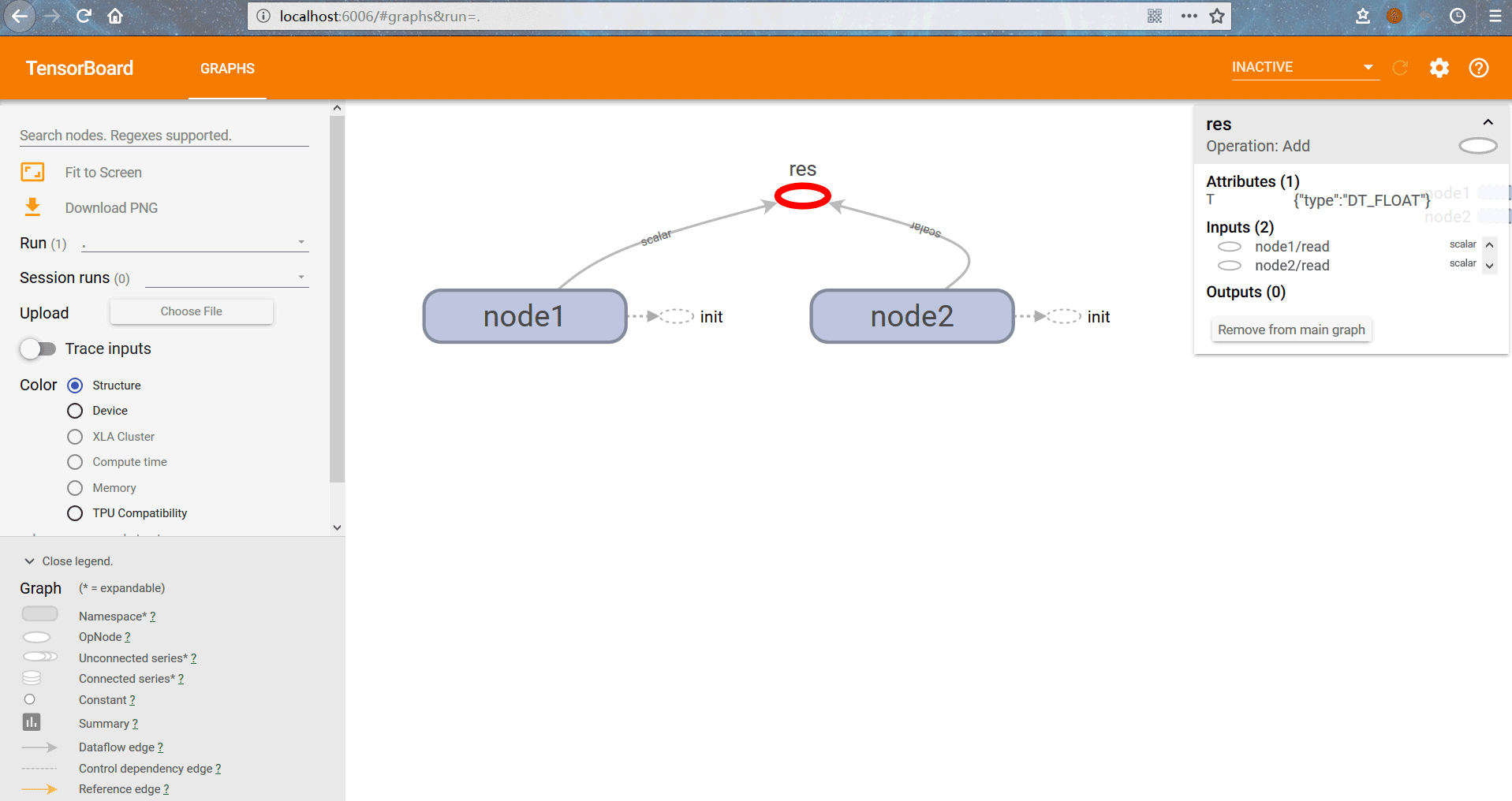```#TensorBoard使用
#定义日志保存位置
log_dir='D:\Temp\MachineLearning\TensorLog'
#显示图片
image_input=tf.reshape(x,[-1,28,28,1])
tf.summary.image('input',image_input,10)  #一次最多显示图片数：10
#显示直方图
tf.summary.histogram('Y',Y3)
#显示标量loss
tf.summary.scalar('loss',loss_function)
tf.summary.scalar('accurancy',accuracy)
#定义汇总summary操作
merge_op=tf.summary.merge_all()

ss=tf.Session()
ss.run(tf.global_variables_initializer())
#定义writer
writer=tf.summary.FileWriter(log_dir,ss.graph)

for epoch in range(train_epochs):
for batch in range(batch_num): #分批次读取数据进行训练
xs,ys=mnist.train.next_batch(batch_size)
ss.run(optimizer,feed_dict={x:xs,y:ys})
#执行summary操作并将结果写入日志文件
summary_str=ss.run(merge_op,feed_dict={x:xs,y:ys})

loss,acc=ss.run([loss_function,accuracy],\
feed_dict={x:mnist.validation.images,y:mnist.validation.labels})
print('第%2d轮训练：损失为：%9f，准确率：%.4f'%(epoch+1,loss,acc))```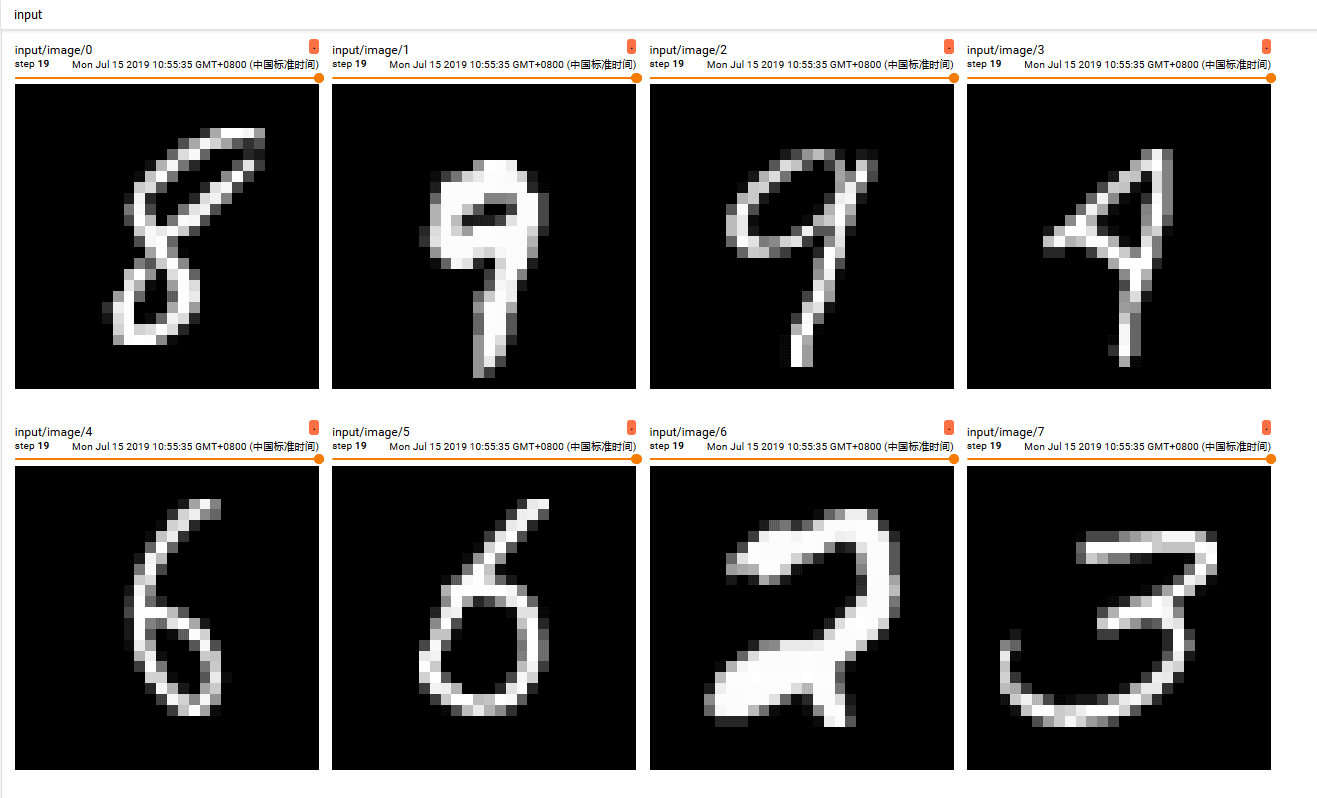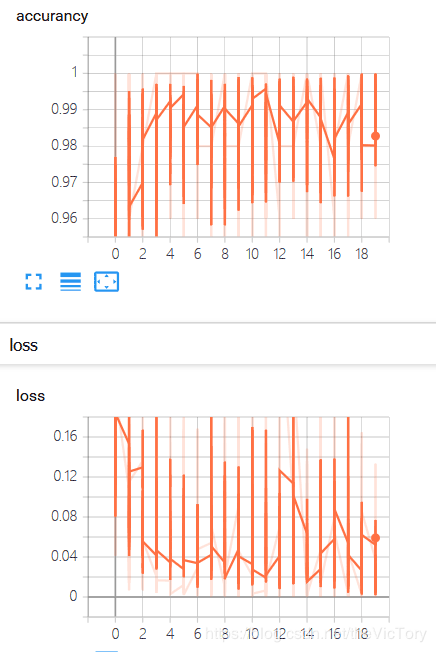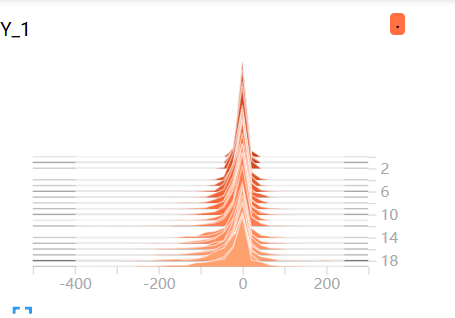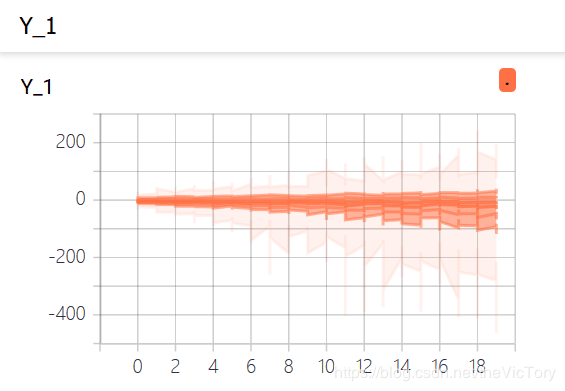#### 您可能感兴趣的文章:

• 本文相关：
• python3.6.3安装图文教程 tensorflow安装配置方法
• python使用tensorflow保存、加载和使用模型的方法
• python使用tensorflow进行图像处理的方法
• 详解python使用tensorflow入门指南
• python tensorflow学习之识别单张图片的实现的示例
• win10下python3.5.2和tensorflow安装环境搭建教程
• python使用tensorflow深度学习识别验证码
• tensorflow平台下python实现神经网络
• python tensorflow基于cnn实现手写数字识别
• python(tensorflow框架)实现手写数字识别系统的方法
• python实现的单向循环链表功能示例
• 浅谈django三种缓存模式的使用及注意点
• 将python运行结果保存至本地文件中的示例讲解
• python多线程高级锁condition简单用法示例
• python简直是万能的，这5大主要用途你一定要知道！（推荐）
• python实现五子棋人机对战游戏
• 记录django开发心得
• python环境下搭建属于自己的pip源的教程
• python断言assert的用法代码解析
• python模拟随机游走图形效果示例
• 免责声明 - 关于我们 - 联系我们 - 广告联系 - 友情链接 - 帮助中心 - 频道导航